# Weighted Average Cost Method

The use of a weighted average to determine the amount that goes into COGS and inventory

## What is Weighted Average Cost (WAC)?

In accounting, the Weighted Average Cost (WAC) method of inventory valuation uses a weighted average to determine the amount that goes into COGS and inventory. The weighted average cost method divides the cost of goods available for sale by the number of units available for sale. The WAC method is permitted under both GAAP and IFRS accounting.

### Weighted Average Cost (WAC) Method Formula

The formula for the weighted average cost method is as follows: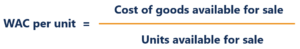Where:

• Costs of goods available for sale is calculated as beginning inventory value + purchases.
• Units available for sale are the number of units a company can sell or the total number of units in inventory.

### Understanding Costs of Goods Available for Sale

The bundling of costs is referred to as the cost of goods available for sale. The costs of goods available for sale are either allocated to COGS or ending inventory. Allocating the costs of goods available for sale is referred to as a cost flow assumption. There are several cost flow assumptions such as:

1. FIFO (first-in, first-out)
2. LIFO (last-in, first-out)
3. WAC (weighted average cost)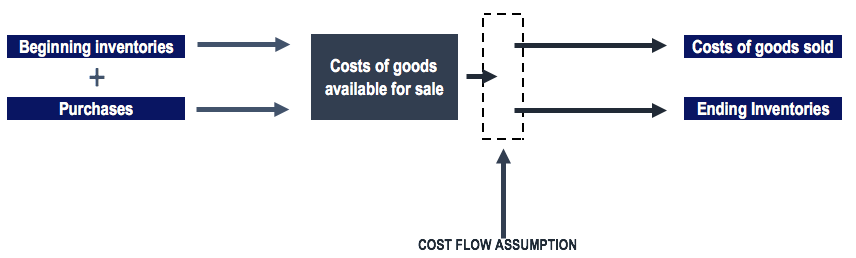### The WAC Method under Periodic and Perpetual Inventory Systems

Using the weighted average cost method yields different allocation of inventory costs under a periodic and perpetual inventory system.

In a periodic inventory system, the company does an ending inventory count and applies product costs to determine the ending inventory cost. COGS can then be determined by combining the ending inventory cost, beginning inventory cost, and the purchases throughout the period.

A perpetual inventory system keeps continual tracking of inventories and COGS. The perpetual inventory system provides more information for the management of inventory levels. However, this method of inventory tracking can be costly for a company. In a perpetual inventory system, the weighted average cost method is referred to as the “moving average cost method”.

Below, we will use the weighted average cost method and identify the difference in the allocation of inventory costs under a periodic and perpetual inventory system.

### Example of the WAC Method

A company, at the beginning of its January 1 fiscal year, reported a beginning inventory of 300 units at a cost of \$100 per unit. Over the first quarter, the company made the following purchases:

• January 15 purchase of 100 units at a cost of \$130 = \$13,000
• February 9 purchase of 200 units at a cost of \$150 = \$30,000
• March 3 purchase of 150 units at a cost of \$200 = \$30,000

• End of February sales of 100 units
• End of March sales of 70 units

Under the periodic inventory system, we would simply determine the cost of goods available for sale and the units available for sale at the end of the first quarter: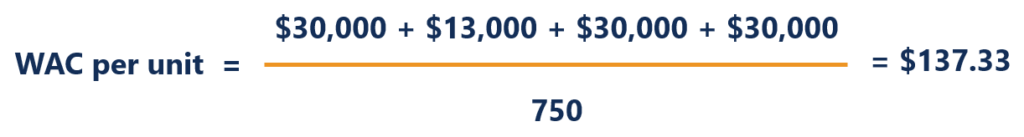For the sale of 170 units over the January-March period, we would allocate \$137.33 per unit sold. The rest would go into ending inventory. Therefore:

• 170 x \$137.33 = \$23,346.10 in COGS
• \$103,000 – \$23,346.10 = \$79,653.90 in ending inventory

Note: The numbers may be slightly off due to rounding off.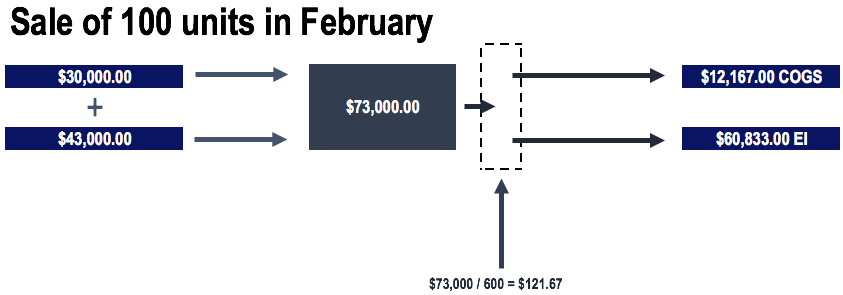Under the perpetual inventory system, we would determine the average before the sale of units.

Therefore, prior to the sale of 100 units in February, our average would be: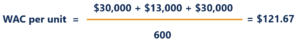For the sale of 100 units in February, the costs would be allocated as follows:

• 100 x \$121.67 = \$12,167 in COGS
• \$73,000 – \$12,167 = \$60,833 remain in inventory

Note: The numbers may be slightly off due to rounding off.

Prior to the sale of 70 units in March, our average would be: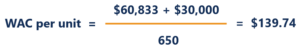For the sale of 70 units in March, the costs would be allocated as follows:

• 70 x \$139.74 = \$9,781.80 in COGS
• \$90,833 – \$9,781.0 = \$81,051.20 in ending inventory

Note: The numbers may be slightly off due to rounding off.

The diagrams would look as follows under the perpetual inventory system: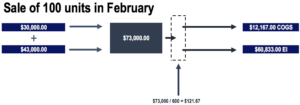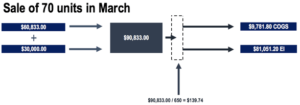### Comparing the WAC Method under the Periodic and Perpetual Inventory Systems

Comparing the costs allocated to COGS and inventory, we can see that the costs are allocated differently depending on whether it is a periodic or perpetual inventory system. However, notice that the total costs remain the same (as they should).

In our example, the inventories purchased experienced a price appreciation. January purchase costs per unit were \$130, February purchase costs per unit were \$150, and March purchase costs per unit were \$200. Therefore, since the periodic system uses the costs of goods available for sale over the entire quarter, more is allocated to the costs of goods sold for the sale of inventory.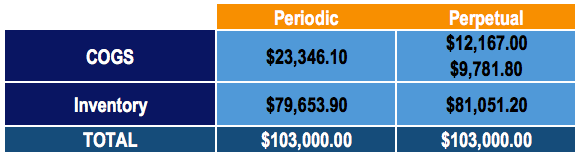CFI offers the Financial Modeling & Valuation Analyst (FMVA)™ certification program for those looking to take their careers to the next level. To keep learning and advance your career, the following CFI resources will be helpful:

• Days Inventory Outstanding (DIO)
• Inventory Turnover
• Cost of Goods Manufactured (COGM)
• Financial Accounting Theory